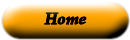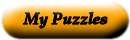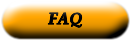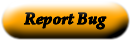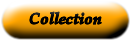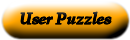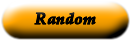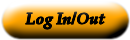### Chapter 4 Vocabulary Match

Vocabulary terms for Common Core Algebra 1: An Introduction to Functions

 1 Arithmetic Sequenc _____ A relation that assigns exactly one value in the range to each value in the domain. 2 Common Difference _____ Any set of ordered pairs. 3 Continuous Graph _____ A function whose graph is a line. This can be represented with a linear equation. 4 Dependent Variable _____ A method used to determine if a relation is a function or not. 5 Discrete Graph _____ The difference between consecutive terms of an arithmetic sequence. 6 Domain _____ A variable that provides the output values of a function. 7 Explicit Formula _____ The possible values of the output, or dependent variable, of a relation or function. 8 Function _____ To write a rule in this formate, you use the symbol f(x) in place of y. 9 Function Notation _____ An ordered list of numbers that often forms a pattern. 10 Input _____ A value of the dependent variable. 11 Independent Variable _____ The possible values for the input of a relation or function. 12 Linear Function _____ This type of formula defines the terms in a sequence by relating each term to the ones before it. 13 Nonlinear Function _____ A graphy compose of isolated points. 14 Output _____ A number sequence formed by adding a fixed number to each previous term to find the next term. 15 Range _____ This formulat expresses the nth term of a sequence in terms of n. 16 Recursive Formula _____ A value of the independent variable. 17 Relation _____ A variable that provides the input values of a function. 18 Sequence _____ A function whose graph is not a line nor part of a line. 19 Term of Sequence _____ A graph that is unbroken. 20 Vertical Line Test _____ A reference to any number in a sequence.

Use the "Printable HTML" button to get a clean page, in either HTML or PDF, that you can use your browser's print button to print. This page won't have buttons or ads, just your puzzle. The PDF format allows the web site to know how large a printer page is, and the fonts are scaled to fill the page. The PDF takes awhile to generate. Don't panic!Web armoredpenguin.com